# 如何在声学仿真中根据频带自动划分网格

2019年 6月 26日

### 自由场有限元波动问题简介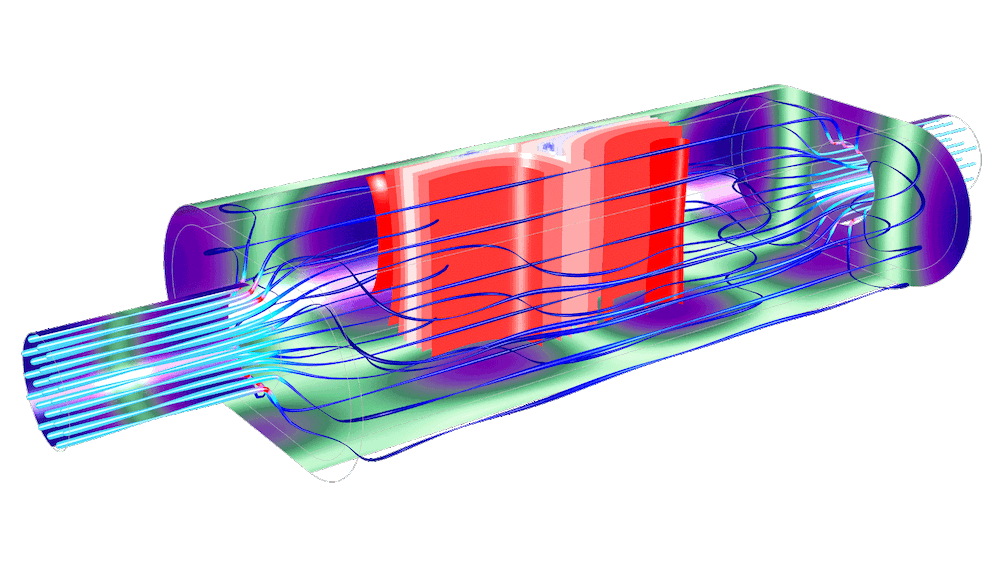### COMSOL Multiphysics® 中的高效网格

#### 单倍频程网格的设置

f_{C,n} = 2^n \times f_0

f_L = 2^{-\frac
{1}{2}} \times f_{C,n}
,

f_U = 2^{\frac{1}
{2}} \times f_{C,n}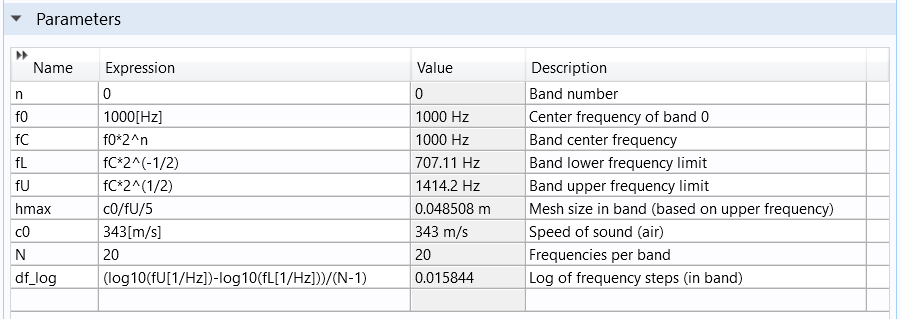10^{\textrm
{range}
(\log_{10}(f_L), df_\textrm{log}, \log_{10}(f_U) – df)}
,hmax = 343[m/s]/f_U/5.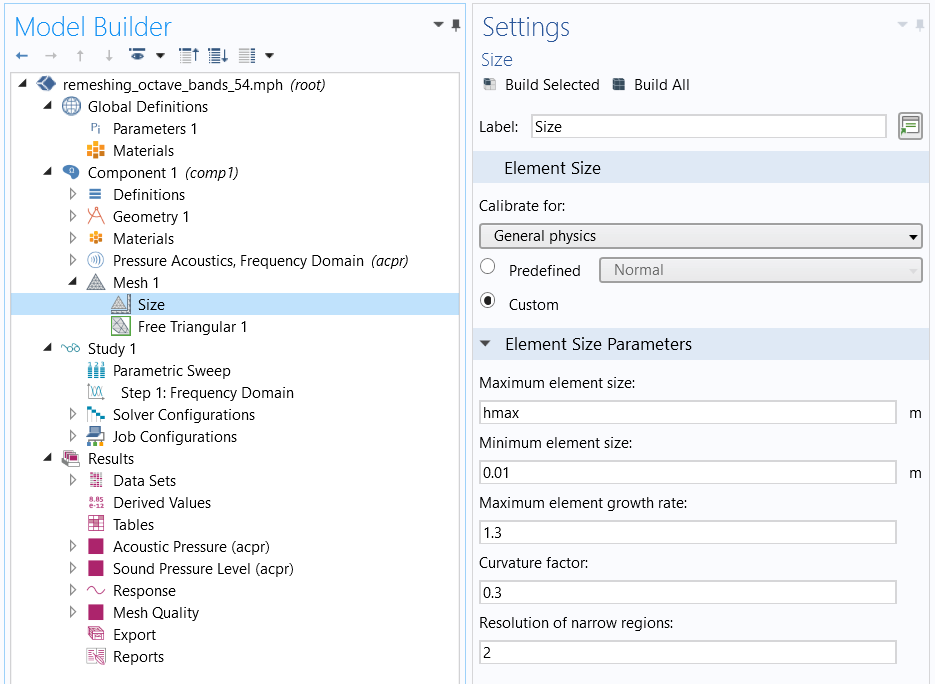#### 多个倍频带的设置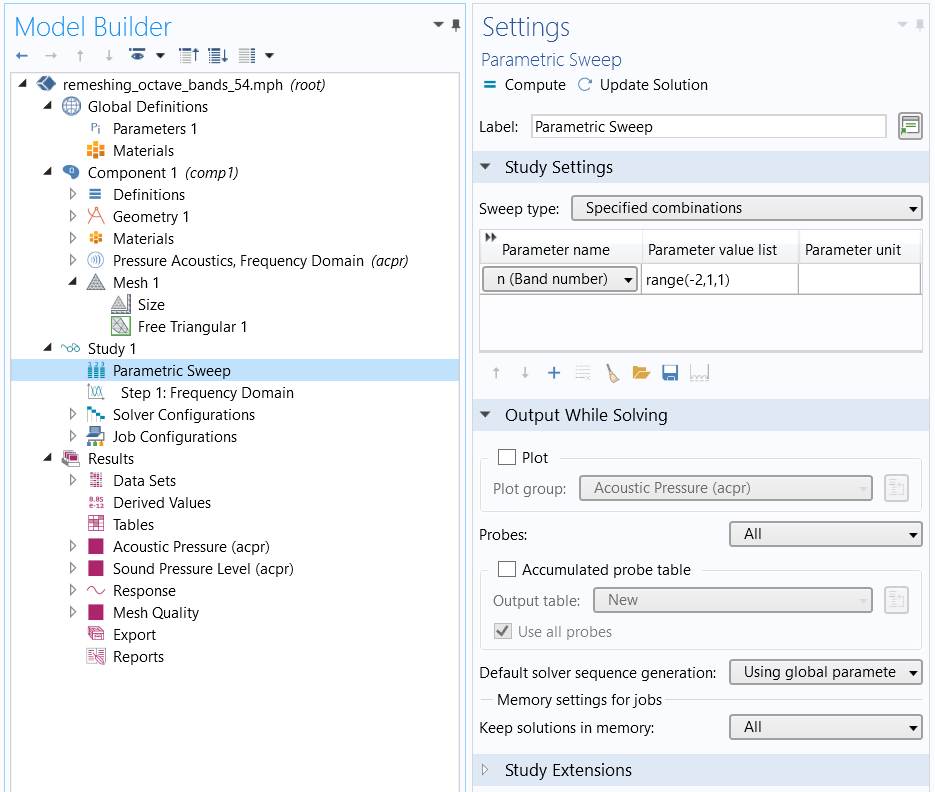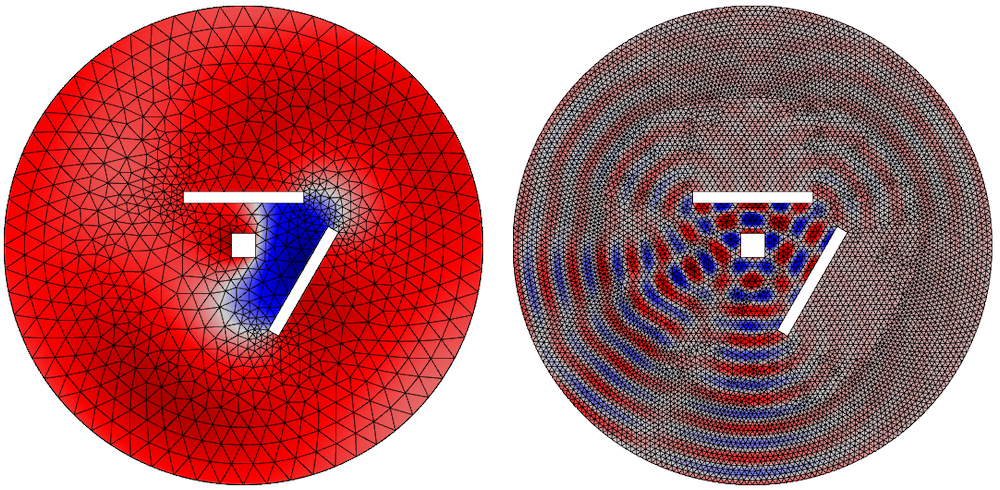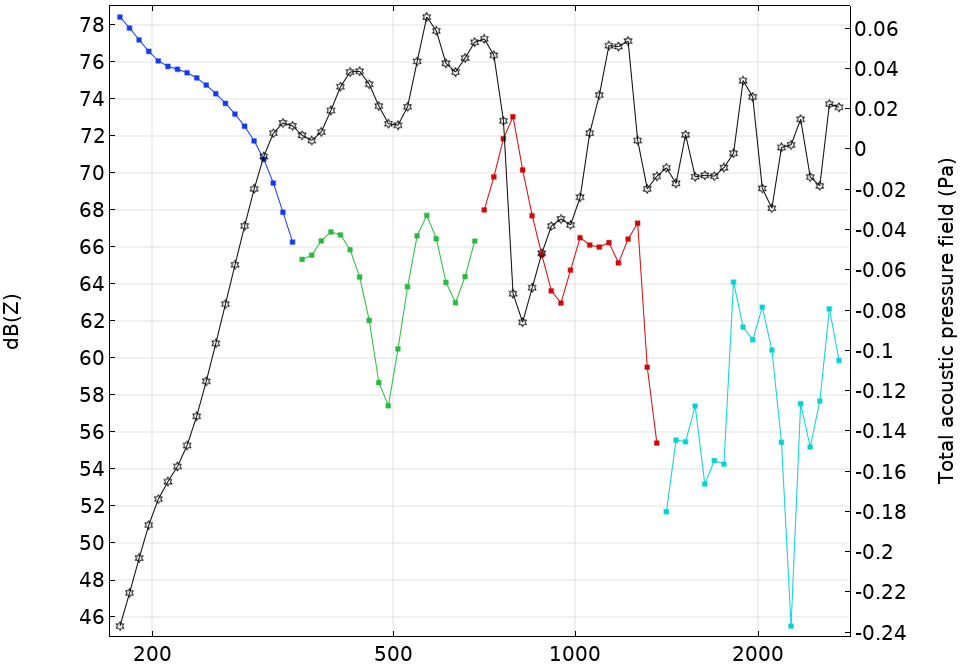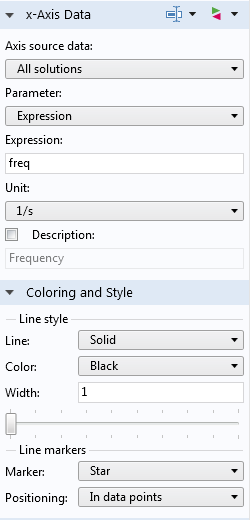#### 第 n 个倍频带的设置

f_{C,n} = 2^\frac{n}
{b}
\times f_0

f_L = 2^{-\frac
{1}{2b}} \times f_{C,n}
,

f_U = 2^{\frac{1}
{2b}} \times f_{C,n}
,